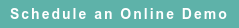# RoyaltyStat Blog

### Posts by Topic

In transfer pricing, a frequent “comparability adjustment” to (gross or operating) profits involving inventories is spurious. The OECD is wrong disseminating misconceived guidance about “working capital” adjustment that includes inventories. The inventory adjustment to profits is bogus because inventory is included in cost of goods sold (COGS); thus, the same inventory variable appears on both sides of the adjustment equation, making the proposed adjustment false. Follow at your peril: OECD, Comparability Adjustments (July 2010), ¶¶ 16-17: http://www.oecd.org/tax/transfer-pricing/45765353.pdf

The IRS has published similar argy-bargy: https://www.irs.gov/pub/irs-apa/study_guide_exhibit_d.pdf

#### Transposing Inventory to the Right-Side

Unless inventory is moved from two sides and collected on the right-side of the equation, the OECD sponsored “working capital” adjustment is indefensible. To see this fake adjustment, let’s define:

##### (1)  COGS = Purchases + V(t) – V(t – 1),

where V(t) denotes inventory at the end of the current year, and V(t – 1) denotes inventory at the beginning of the year.

Also, let's define two-year average inventory:

##### (3)  Gross Profits = α + β 0.5 {V(t) + V(t – 1)},

where the intercept (α) represents adjusted gross profits and the regression coefficient (β) is regarded by the OECD as “working capital adjustment is an attempt to adjust for the differences in time value of money between the tested party and potential comparables, with an assumption that the difference should be reflected in profits.” OECD, ibiden, ¶ 16. Emphases added. The OECD arbitrarily assigns a negative sign to the regression coefficient. We shall leave aside the untenable assumption that the regression coefficient (β) represents an interest rate, and that imputed inventory financing costs can be regarded as an operating expense.

We know that gross profits are defined as Net Sales minus COGS, and so we substitute (1) into (3) to reveal the OECD naive transgression:

##### (5)  Net Sales – {Purchases + V(t) – V(t – 1)} = α + β 0.5 {V(t) + V(t – 1)},

and it becomes obvious that inventory is found on both sides of the equation, rendering use of (3) or (5) an infelicitous economics breach.

A correct way to test if gross profits (or operating profits after subtracting operating expenses from gross profits) is influenced by inventory, and thus if an adjustment is defensible, is to transpose inventory from the left to the right side of (5):

##### (8) Adjusted Gross Profits = α + μ1 V(t) − μ2 V(t – 1),

where Adjusted Gross Profits = (Net Sales – Purchases),  μ1 = (1 + β 0.5), and μ2 = (1 − β 0.5).

The dependence of these two regression coefficients (μ1 and μ2is disturbing.

Equation (8) reveals that the OECD “working capital” adjustment equation (3) or (5) is wrong and should be abandoned. The corrected inventory adjustment equation (8) has merit because adjusted gross profits are free of inventory. However, this adjustment equation (8) is valid (empirically defensible) only if each regression coefficient (μ1 and μ2), tested against contemporaneous profit and inventory data from the selected comparables, shows statistical significance using accepted t-statistics. In practice, this means that the t-statistics of the regression coefficient of each independent variable (end of current year and one-year old inventory), which is the ratio of the estimated regression coefficient divided by its standard error, is greater than 2. In addition, the regression residuals must conform to the ordinary least squares (OLS) assumption that they are random (residuals must show no discernible pattern) and exhibit constant variance. These are expert orders to follow before inventory adjustment can gain acceptability.

If we modify (3) to consider inventory at the end of the year, and not an average of two years, then the change is trivial. Equation (3) becomes Gross Profits = α + β V(t)  and the adjustment equation (8) becomes Net Sales – Purchases = α + ( 1 + β) V(t) − V(t – 1).